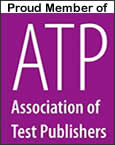UPDATED 2020

# The Math Test Guide

The Math Test Summary
What: Tests for required classes in elementary, middle, high school and college.
Who: Students of any grade level.
Where: Local public school system or university.
When: Multiple times a year.
How: Paper and pencil.
Type: Basic math, algebra, pre-algebra, calculus, statistics, trigonometry, and geometry.
Why: Required in all grade levels by public school systems.
Time: Varies.
Language: English
Preparation: Workbooks, flash cards and tutoring all can be beneficial.
Cost: Free

By Alyssa Choiniere, Tests.com Contributing Writer

Mathematics, for many, is the subject causing the most despondence in an academic career, and those who understand it are often the subject of great envy. Yet someone must have discerned long ago that this was a fundamental study in life, for it is a focal point of teachers beginning in kindergarten, continuing in elementary, middle school and high school, and even making an appearance on the list of required courses in college.

To understand math and survive high school and college courses, it is essential to understand the basics in elementary school and middle school. If you, or your child, are having difficulties with math, there are endless resources available for studying this notorious subject in any grade level. So fear not. As Albert Einstein once said, “Do not worry about your difficulties in mathematics. I assure you that mine are greater.”

Math in School

Most public school systems follow the same general timeline for teaching math:

• Kindergarten – Simple counting problems, basic addition and basic subtraction
• 3rd Grade – Decimals, multiplying decimals and multiplying fractions
• 4th Grade – Fundamentals of equations
• High School – Algebra II as a graduation requirement, and also calculus, statistics, trigonometry and other electives
• College – At least one mathematics course is required for graduation; non-math related majors might take college algebra, general math, business math or statistics.

Types of Math Tests

In the earlier years of math, students will be tested on addition, subtraction, multiplication, division, and long division and multiplication. A test problem for students in early middle school, or late elementary school, may look like this:

8383

X 346

After elementary school, pre-algebra will instruct students in properties of integers, rounding, place value, adding and subtracting decimals, multiplication and division of decimals, percentages, exponents, ratios, proportions, addition and subtraction of fractions, multiplication and division of fractions, square roots, distance, rates and time. For example, 6 ½ x ½.

Geometry is the study of space, when students learn about classifying angles, intersecting lines, circles, congruent figures, similar figures, polygons, areas, 3-dimensional figures, and the Pythagorean theorem. The following is a problem using the formula for circles:

A circle has a radius of 2.4 cm. What is its circumference?

In algebra, students learn the order of operations to assist in learning equations. They then learn how to perform equations, inequalities and two-step equations and inequalities, such as 3+b>56.

Statistics courses involve data. Problems involve the interpretation, collection and analysis of that data through mathematical means. An example involving standard deviation is:

The average number of people on the Internet at any given time under the age of 30 is 364,643, with a standard deviation of 34,456. The average number of people on the Internet over the age of 30 is 136,987, with a standard deviation of 12,986. In a nationwide survey, suppose 100,000 were questioned on their Internet habits. What is the probability that the number of people currently online under the age of thirty is at least twice that of the number of people over age 30?

Calculus is the study of change. It involves limits, derivatives, integrals and infinite series. For example:

Using the limit definition, complete the derivative f(x) for f(x)= 2/3x-4/5.

Trigonometry is the study of triangles. It is based around the functions sine (sin), cosine (cos) and tangent (tan). A test question may look like this:

If the distance of a tree from a building is 250 m, and the angle subtended by the top of the building with the ground is 20 degrees, what is the height of the building in meters?

How to Study

For students having trouble math, there are many resources available to help out.

• Flashcards – These are an excellent way to memorize basic math facts, such as addition, subtraction, multiplication tables and formulas.
• Tutoring – Many schools offer math tutoring programs. Tutoring is often offered both by teachers and peers. Tutoring is also offered by private firms and individuals, either in home or at a learning center.
• Textbooks – Many student math textbooks include additional problems and answers in the back of the book, separate from material taught in class. Students can spend extra time working on these problems to hone their math skills.
• Workbooks – Textbooks can be supplemented by workbooks, which are full of tips, tricks and extra problems. This kind of additional practice can be purchased by grade level or by particular subject area.

Do you or your child need additional math help? Check out our Math Test Directory to find the perfect study aids.

Sources: Math.com; National School Boards Association - nsba.org